# 1. 主成分分析

まずは主成分分析をしてみる。次のcolaboratryを参照してほしい。

https://colab.research.google.com/drive/1Te2yuuMpqYy_UkQxinWAAZ-kxtcPVZpL?usp=sharing

ワインのデータから、 'Color intensity', 'Flavanoids', 'Alcohol', 'Proline'のデータについて、scikit-learnのPCAモジュールを用いて主成分分析を行っている。

なお、主成分分析とデータについては主成分分析を Python で理解するを参照した。

colaboratryの1章で、主成分分析をしてbiplotを実行している。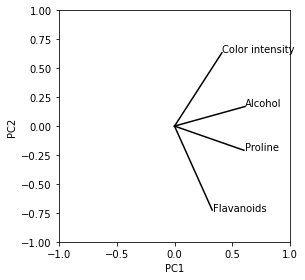wineデータの4変数についてのbiplot

また、各変数の相関係数は次のようになった。

Color intensity Flavanoids Alcohol Proline
Color intensity 1.000000 -0.172379 0.546364 0.316100
Flavanoids -0.172379 1.000000 0.236815 0.494193
Alcohol 0.546364 0.236815 1.000000 0.643720
Proline 0.316100 0.494193 0.643720 1.000000

このbiplot上の変数同士の角度と、相関係数にはなにか関係があるだろうか？例えば、角度が0度に近ければ相関が高く、90度近ければ相関が低いと言えるだろうか？

colaboratryの2章で相関係数とbiplotの角度の$\cos$についてプロットしてみている。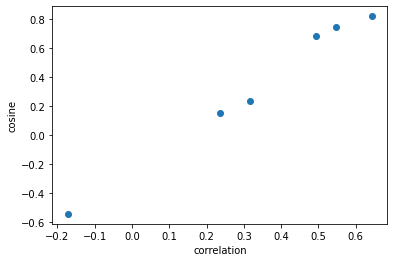相関係数とbiplotの角度の$\cos$の関係

# 2. 分散共分散行列と相関行列の関係

データ数$n$の2種類のデータ$x,y$をどちらも平均$0$、不偏分散を$1$に標準化しておく 相関係数$r _ {xy}$は次のように変形できる。

\begin{aligned}r_{xy}&=\frac{\Sigma(x-\bar{x})(y-\bar{y})}{\sqrt{\Sigma(x-\bar{x})^2}\sqrt{\Sigma(y-\bar{y})^2}}\\&=\frac{\Sigma(x-\bar{x})(y-\bar{y})}{n-1}\left/\left[\sqrt{\frac{\Sigma(x-\bar{x})^2}{n-1}}\sqrt{\frac{\Sigma(y-\bar{y})^2}{n-1}}\right]\right.\\&=s_{xy}\end{aligned}

となる。ただし$s _ {xy}$は不偏共分散である。

s_{xy}=\frac{\Sigma(x-\bar{x})(y-\bar{y})}{n-1}

よって、標準化された、$m$種類のデータについて$n$個観測した、nm列のデータXを次のように計算した不偏分散共分散行列は相関係数行列と等しくなる。 \Sigma = \frac{X^T X}{n - 1} # 3. 主成分分析と固有値分解 主成分分析は分散共分散行列を固有値分解することでできる。 \begin{aligned}\Sigma &= V_{pca} L V^T_{pca}\\V_{pca} &= \left( \begin{array}{cccc}\pmb{v}_1 & \pmb{v}_2 & \cdots & \pmb{v}_m\end{array} \right)\\&=\left( \begin{array}{c}\pmb{u}_1^T \\\pmb{u}_2^T \\\vdots \\ \pmb{u}_m^T\end{array} \right)\\L &= \left( \begin{array}{cccc}\lambda_1 & 0 & 0 & \cdots & 0\\0 & \lambda_2 & 0 & \cdots & 0\\\vdots & & \ddots & & \vdots\\\\0 & 0 & & \cdots & \lambda_m\end{array} \right)\end{aligned} この時、固有値は大きい順に並び替えてあるものとする。 そして、元のデータを主成分に変換したい場合はXV _ {pca}を計算する。 # 4. 寄与度プロット(biplot)の角度と相関係数 PCAでは、第一主成分と第二主成分における観測変数の寄与度をプロットするbiplotによって、データ全体の傾向を掴もうとすることがよく行われる。そのときの寄与度プロットでのi列目とj列目の観測変数の矢印同士の角度を\theta _ {ij}とすると、その余弦\cos{\theta _ {ij}}はベクトル\pmb{u} _ i\pmb{u} _ jの第1成分、第2成分を成分としたベクトル\pmb{u}' _ i\pmb{u}' _ j内積\|\pmb{u}' _ i\|\|\pmb{u}' _ j\|で割ったものとなる。つまり、 \begin{aligned}\cos{\theta_{ij}}&=\frac{\pmb{u}'_i\cdot\pmb{u}'_j}{\|\pmb{u}'_i\|\|\pmb{u}'_j\|}\\&=\frac{u_{i1}u_{j1}+u_{i2}u_{j2}}{\sqrt{(u_{i1}^2+u_{j1}^2)(u_{i2}^2+u_{j2}^2)}}\end{aligned} 一方、i列目とj列目の変数の相関係数 \begin{aligned}r_{ij}&=\pmb{u}_i^TL\pmb{u}_j\\&=(u_{i1},u_{i2},\cdots)\left( \begin{array}{cccc}\lambda_1 & 0 & 0 & \cdots & 0\\0 & \lambda_2 & 0 & \cdots & 0\\\vdots & & \ddots & & \vdots\\\\0 & 0 & & \cdots & \lambda_m\end{array} \right)(u_{j1},u_{j2},\cdots)^T\\&=\lambda_1u_{i1}u_{j1}+\lambda_2u_{i2}u_{j2}+\cdots\end{aligned} である。この2つは等しくない。しかし、もし仮にPC1とPC2の2つの分散がすべての分散のかなりの割合を占めていて、PC3以降の分散が無視できる場合、 \begin{aligned}r_{ij}&\approx\lambda_1u_{i1}u_{j1}+\lambda_2u_{i2}u_{j2}\\&=(\sqrt{\lambda_1}u_{i1},\sqrt{\lambda_2}u_{i2})\cdot(\sqrt{\lambda_1}u_{j1},\sqrt{\lambda_2}u_{j2})^T\\&=\pmb{u}''_i\cdot\pmb{u}''_j\\&=\|\pmb{u}''_i\|\|\pmb{u}''_j\|\cos{\theta'_{ij}}\end{aligned} となる。PC1とPC2における観測変数の寄与度にそれぞれPC1とPC2の標準偏差をかけた座標でプロットした時、つまり(\sqrt{\lambda _ 1}u _ {i1},\sqrt{\lambda _ 2}u _ {i2})(\sqrt{\lambda _ 1}u _ {j1},\sqrt{\lambda _ 2}u _ {j2})などとプロットした時の角度$\theta'$について考える。このプロットの方法を修正biplotと呼ぶことにする。このとき、修正biplotのベクトルの長さ$\|\pmb{u}''\|$がどれもほぼ同じ$(\approx l)$であれば、

r_{ij}\approx l^2\cos{\theta'_{ij}}

となり、$\cos{\theta' _ {ij}}$相関係数$r _ {ij}$と比例する。また、ベクトルの長さが一定以上あり、$\cos{\theta' _ {ij}}$が0であれば、つまりベクトル同士が90度であれば、相関は0といっていい。

# 5. 具体例

## 5.1 ワインデータ

https://colab.research.google.com/drive/1Te2yuuMpqYy_UkQxinWAAZ-kxtcPVZpL?usp=sharing

colaboratryの3章で固有値固有ベクトル、そして分散の割合を確認している。

PC1 2.134122
PC2 1.238082
PC3 0.339148
PC4 0.288648

そして固有ベクトル$V _ {pca}$pca.components_.Tは次のようになっていた。

PC1 PC2 PC3 PC4
Color intensity 0.409416 0.633932 0.636547 -0.159113
Flavanoids 0.325547 -0.725357 0.566896 0.215651
Alcohol 0.605601 0.168286 -0.388715 0.673667
Proline 0.599704 -0.208967 -0.349768 -0.688731

この表の１行それぞれが$\pmb{u}$ベクトルである。

PC1 0.533531
PC2 0.309520
PC3 0.084787
PC4 0.072162

PC1とPC2の分散が全体の約84%の分散を占めている。

また、修正biplotでのベクトルのnormは次のようになっていた

Color intensity 0.924809
Flavanoids 0.936794
Alcohol 0.904300
Proline 0.906416

ベクトルの長さがだいたい同じである。よって、修正biplotの方法でプロットすれば、角度の$\cos$相関係数が多少比例するはずである。

colaboratryの5章で通常のbiplotと修正biplotを比較している。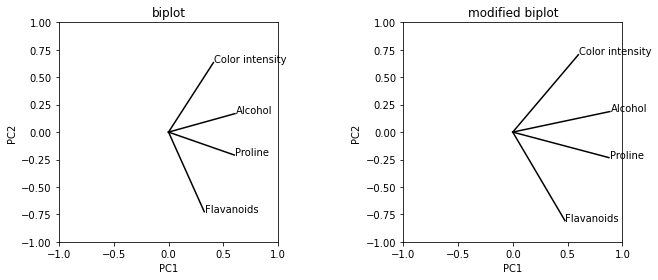PC1の分散がPC2より大きい分、修正biplotでは通常のbiplotに比べて横に引き伸ばされている。

そしてcolaboratryの6章で相関係数と通常のbiplotと修正biplotそれぞれでの角度の$\cos$をプロットしている。修正biplotでは相関係数$\cos$がほぼ比例していることがわかる。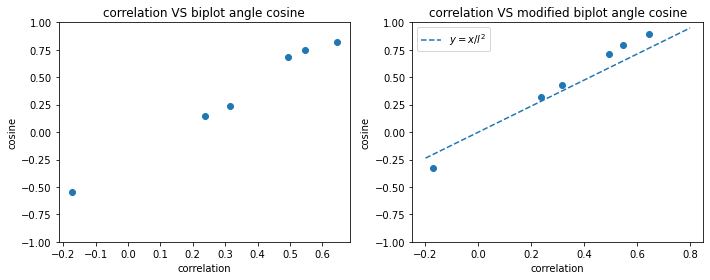## 5.2 すべてのワインデータ

colaboratryのAppendix 2章でワインデータについて13ある全ての観測変数でPCAを行っている。修正biplotは次のようになった。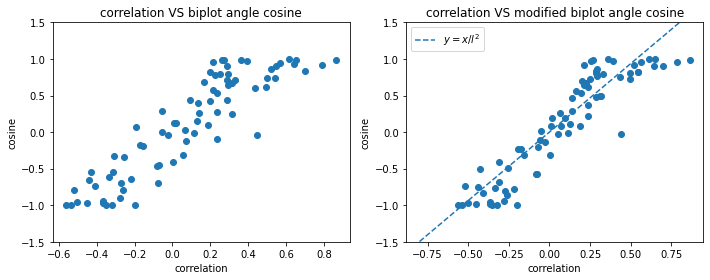このときPC1とPC2の分散が全体の約56%の分散を占めてた。

つまりこの場合、PC1とPC2の分散が全体の大部分を占めていて、修正biplotのベクトルの長さがだいたい同じであるので相関係数と修正biplotの角度の$\cos$がだいたい比例している。

## 5.3 ランダムなデータ

colaboratryのAppendix 3章で観測変数が10あるランダムなデータを生成してPCAを行っている。1変数目、2変数目、3変数目同士、そして4変数目、5変数目、6変数目同士の相関が高くなるようにした。それ以外の相関は低く設定してある。修正biplotは次のようになった。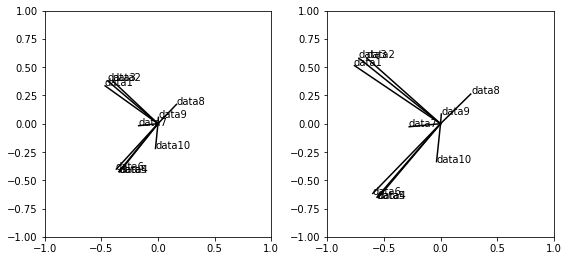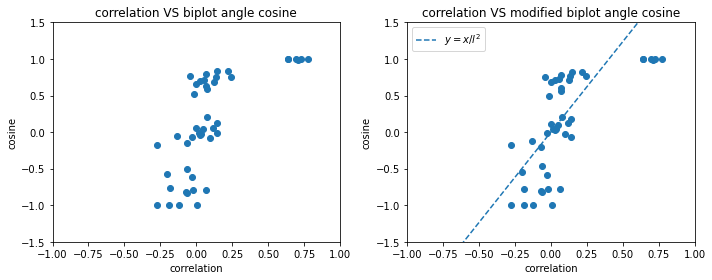このときPC1とPC2の分散が全体の約49%の分散を占めてた。

つまりこの場合は、PC1とPC2の分散が全体の大部分を占めてはいるが、修正biplotのベクトルの長さがばらばらなので相関係数と修正biplotの角度の$\cos$は比例しない。

# 6.結論

• PC1とPC2の分散が全体の大部分を占めていて、修正biplotのベクトルの長さがだいたい同じである場合、相関係数と修正biplotの角度の$cos$はほぼ比例する。
• PC1とPC2の分散が全体の大部分を占めていて、修正biplotのベクトルの長さが少しでもあり、ベクトル同士の角度が90度に近いものは相関は小さい。

# 7. 相関を見たいときは

https://colab.research.google.com/drive/1Ga7XhsNVs7H8zO7W5O--rotPiKxDUBLV?usp=sharing

クラス分類をone-hot encodingにして相関を取り、相関係数の大きさをedgeの太さにしてグラフ化した。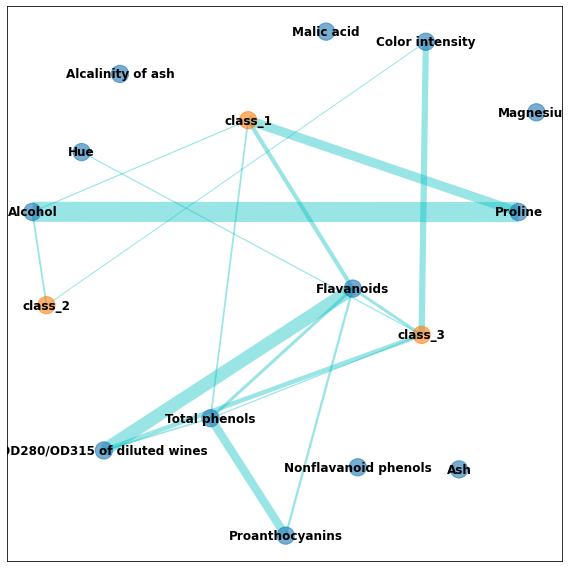# How to install psi4 in a Docker container with a jupyter environmentPsi4: OPEN-SOURCE QUANTUM CHEMISTRY

Psi4 is a Python module that can perform quantum chemistry calculations. This article explain how to compile and install Psi4 in the jupyter/minimal-notebook container.

# How I came to write this article

I tried to install Psi4 in the jupyter/minimal-notebook container. However, as shown in the question in SO, I could not install it due to UnsatisfiableError. What should I do in this case? Psi4 is open source software. The answer was right in front of me. All I had to do was follow the philosophy of OSS. Yes, I should build it myself.

# 1. Configure the resources you provide to docker

Building Psi4 requires a lot of CPU and memory resources, so make sure you provide at least 3 cores and 4GB memory for docker. If you are a Windows user and using docker desktop for Windows, you can set this up in the resources section of the docker desktop setting. However, this setting is only required in Hyper-V mode. Because if you are using WSL 2 backend, these resources are managed by Windows. Windows will allocate resources containers need, when containers need them. Please read the documentation for more details.

# 2. Get familiar with Jupyter Docker Stacks

The jupyter/minimal-notebook I am using here is one of the family of Jupyter Docker Stacks. This docker image provides a jupyter environment. The default user is jovyan, but if you run bash as jovyan, you can't install packages by apt-get because jovyan doesn't have permissions. You can start bash as the root by specifying --user root when you docker run. In addition to this, there are various other settings. Read the documentation to get familiar with them.

# 3. Start bash in the container

Start bash in the container as root.

$docker exec -it [container ID] /bin/bash (base) root$

# 4. Clone Psi4 repository

Clone the Psi4 repository.

(base) root$git clone https://github.com/psi4/psi4.git (base) root$ cd psi4

# 5. Create new conda environment

Start bash as root. Psi4 only supports until python 3.7, so I should create a conda environment for python 3.7.

(base) root$conda create -n quantum python=3.7 (base) root$ conda activate quantum
(quantum) root$ Add a new quantum environment to jupyter so that you can program in the quantum environment. (quantum) root$ conda install ipykernel
(quantum) root$python -m ipykernel install --user --name=quantum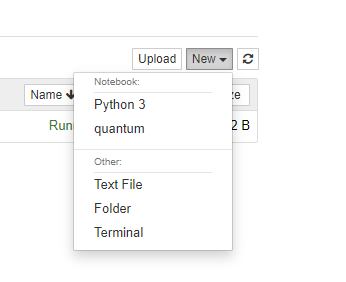add quantum environment to jupyter Please read this article for more details. # 6. Install the packages needed to build Type the following command to install the packages required for the build. Psi4 documentation lists tools and dependencies required for the build. (quantum) root$ apt-get update
(quantum) root$apt-get install -y build-essential cmake clang libssl-dev BLAS and LAPACK libraries are also required. I used openblas. Uh, do you say I should build BLAS too if I follow the OSS philosophy? Shhh! (quantum) root$ apt-get install -y libopenblas-base libopenblas-dev

We will also need numpy, networkx, pint, and pydantic, which we will install with conda.

(quantum) root$conda install -y numpy networkx pint pydantic Although it is not mentioned in the documentaion, if you read CmakeList.txt carefully, you will see that MPFR and Eigen library is also required. Install them as well. (quantum) root$ apt-get install -y libmpfr-dev libeigen3-dev

# 7. Buid and install

It's time to build! Type the following command to Configure and Generate to build.

(quantum) root /psi4$mkdir build (quantum) root /psi4$ cd build
(quantum) root /psi4/build$cmake .. Let's build! (quantum) root /psi4/build$ make -jgetconf _NPROCESSORS_ONLN

getconf _NPROCESSORS_ONLN indicates the number of processors available, i.e., the number of threads available, so this command will build with all available processors. If you are using docker desktop for Windows in Hyper-V mode, this is fine. However, if you use WSL2 backend, Windows will try to allcate all processors your PC has to build, which causes systemc instability and memory shortage. It is better to use half the number of all processors, i.e. the number of cores. I am using docker desktop with WSL2 backend and my PC has 8 cores and 16 processors, so I typed the following command.

(quantum) root /psi4/build$make -j8 This build takes several hours. After build is complete, type the following command to install it. (quantum) root /psi4/build$ make install

# 8. Specify install directry as python import path

In the default configuration, the binaries, includes, libraries, etc. should be installed in /usr/local/psi4. In order to use Psi4 as python module, it is needed to append /usr/local/psi4/lib to the python import path. You can do this by appending the directry to sys.path each time as follows.

import sys
sys.path.append("usr/local/psi4/lib")
# you can import psi4 from now
import psi4


Here is another way to simplify your Python code. Make psi4.pth file in /opt/conda/envs/quantum/lib/python3.7/site-packages and specify /usr/local/psi4/lib into it.

(quantum) root $cd /opt/conda/envs/quantum/lib/python3.7/site-packages (quantum) root /opt/conda/envs/quantum/lib/python3.7/site-packages$ vi psi4.pth
### specify /usr/local/psi4/lib into psi4.pth and save. ###

If you do this, you can import Psi4 anyweher in the quantum environment.

# 9. Enjoy quantum chemistry calculations!

If you import Psi4 successfully, you can run the following code and calculate the energy of a watar molcule. Enjoy!

import psi4

h2o = psi4.geometry("""
O
H 1 0.96
H 1 0.96 2 104.5
""")

psi4.energy('scf/cc-pvdz')

>>-76.02663273508807

# 単位の約分と単位の換算について

## 単位の約分について

\begin{aligned}\frac{\cancel\mathrm{mol}*\mathrm{J\cdot \cancel{K^{-1}}\cdot \cancel {mol^{-1}}}*\cancel\mathrm{K}}{\mathrm{m^3}}&=\frac{\mathrm{N\cdot m}}{\mathrm{m^3}}\\&=\mathrm{Pa}\end{aligned}

と単位の約分ができて、単位部分も含め辻褄が合っています。このように、文字式に単位も含め数値を代入することや、単位は約分できることは中学の物理や化学で最初で教えられることだと思います。

## 単位の換算について

1=7.52\times 10^{-3}\ \mathrm{mmHg\cdot Pa^{-1}}

とできるはずです。このとき左辺の$1$には単位はありません。先程の、$p=2.39\times 10 ^ 6\ \mathrm{Pa}$という式の両辺にこの式をかければ、

\begin{aligned}p&=2.39\times 10^6\ \mathrm{Pa}*7.52\times 10^{-3}\ \mathrm{mmHg\cdot Pa^{-1}}\\&=1.80\times10^{4}\ \mathrm{mmHg}\end{aligned}

$\mathrm{mmHg}$に換算できます。このように、「1=数字 ［換算後の単位/換算前の単位］」という式を両辺に掛け、単位の約分をすることで単位の換算ができるようになりいます。

## 3つの単位に関する換算

\frac{\mathrm{atm}}{\mathrm{mmHg}}=760

という式を作ります。このときも右辺には単位はありません。これを$1\ \mathrm{mmHg}=1.33\times10 ^ 2\ \mathrm{Pa}$の両辺に掛けると

\begin{aligned}1\ \cancel\mathrm{mmHg}*\frac{\mathrm{atm}}{\cancel\mathrm{mmHg}} &= 1\ \mathrm{atm}\\&=1.33\times10^2\ \mathrm{Pa}*760\\&= 1.01\times10^5\ \mathrm{Pa}\end{aligned}

$\mathrm{atm}$$\mathrm{Pa}の関係式が得られます。このように3つの単位に関する換算でも単位の約分をうまく使うと、見通しよく換算できると思います。 ## まとめ 「1=数字 ［換算後の単位/換算前の単位］」という関係式をいろいろ変形して使うと、見通しよく換算できることが分かりました。ここで挙げた例は単純なので、このような計算をしなくてもすぐに換算できると思いますが、複雑な単位の換算をしなければいけないときは役に立つと思います。 # Markdownの数式をKaTeXで高速で表示できるようにした いままで作ってきた の変換プログラムをKaTeXに対応させました。はてなブログにそのままTeXの数式を渡すと描写にMathJaxを使うようで、描写がかなり遅いです。数式をKaTeXで変換するようなJavaScriptを入れることで、だいぶ表示を高速化できました。 ### Markdown→はてな記法html ### Markdown→はてな流Markdown ## 使い方 どちらもmd_parserフォルダ内のmd_parser.pyを次のようにインポートして使います。 from md_parser import md_parser md_parser.parse_md_to_hatena(pathlib.Pathオブジェクト,style="default")  styleでスタイルを指定できます。 ## 参考サイト 以下のサイトを参考にさせていただきました。ありがとうございました。 KaTeXのテスト - 七誌の開発日記 はてなブログのキーワード自動リンクを停止する - 0番染色体 # ボルツマン因子と正規分布、ガンマ分布、マクスウェル分布の関係 マセマの「統計力学 キャンパスゼミ」で統計力学を勉強中なのですが、この本にはマクスウェル分布が出てきません。ただ、少し考えるとボルツマン因子e ^ {-E/kT}からマクスウェル分布が出せました。そこで、ボルツマン因子、マクスウェル分布、統計学正規分布、ガンマ分布の関係を整理してみます。 # 統計学 まず統計の知識を整理します。「久保川達也・現代数理統計学の基礎」を参考にしました。 ## 分布関数と確率密度関数 確率変数X分布関数F _ X(x)確率密度関数f _ X(x)=\frac{d}{dx}F _ X(x)と書きます。この記事では確率変数を大文字、その実現値を小文字で書くことにします。 ## 変数変換 確率変数X$$Y=g(X)$と変換したとき、Yの確率密度関数$f _ Y(y)$

f_Y(y)=\frac{d}{dy}F_Y(y)=\frac{d}{dy}P(X\in{x|g(x)\le y})

から導かれます。特に、$g(\cdot)$が単調増加、あるいは単調減少関数の場合は次で与えられます。

f_Y(y)=f_X(g^{-1}(y))\frac{1}{|g'(g^{-1}(y))|}

## 平方変換

f_Y(y)=\left[f_X(\sqrt{y})+f_X(-\sqrt{y})\right]\frac{1}{2\sqrt{y}}

2つの確率変数の組$(X,Y)$$(x,y)周辺となる確率は同時確率密度関数f _ {X,Y}(x,y)を用いて表します。X単体、Y単体の確率密度関数周辺確率密度関数と呼ばれ次のように表します。 f_X(x)=\int_{-\infty}^\infty f_{X,Y}(x,y) dy ## 確率の独立性 同時確率密度関数がそれぞれの確率密度関数の積で表せるとき、独立であると言います。次の場合X$$Y$は独立です。

f_{X,Y}(x,y)=f_X(x)f_Y(y)

# ボルツマン因子とマクスウェル分布の関係

f(\pmb{q},\pmb{p})=\frac{e^{-E/kT}}{\int\int_{-\infty}^\infty e^{-E/kT} d\pmb{q} d\pmb{p} }

## $x$方向にしか動かない1自由粒子の場合

E=\frac{1}{2m}p_x^2

これをボルツマン因子の式に代入すれば、係数を$A$として確率密度関数は次のように表せます。

f(p_x)=A\exp{\left[-\frac{p_x^2}{2mkT}\right]}

E=\frac{1}{2m}\left(p_x^2+p_y^2\right)

これをボルツマン因子の式に代入すれば、係数を$A$として$(p _ x,p _ y)$の同時確率密度関数は次のように表せます。

\begin{aligned}f(p_x,p_y)&=A\exp{\left[-\frac{p_x^2+p_y^2}{2mkT}\right]}\\&=\sqrt{A}\exp{\left[-\frac{p_x^2}{2mkT}\right]}\sqrt{A}\exp{\left[-\frac{p_y^2}{2mkT}\right]}\end{aligned}

f_X(x|1,\frac{1}{a})=\frac{1}{\Gamma(1)}a(ax)^{1-1}e^{-ax}=ae^{-ax}

となります。($\cdots(a2)$)

$(a1)$$S=X ^ 2+Y ^ 2と変数変換したときのS確率密度関数が、式(a2)に一致すれば、(※)は正しいとしてよさそうです。 そこで多変数の変数変換をします。S=X ^ 2+Y ^ 2$$T=X$とすると、x=ty=\pm\sqrt{s-t ^ 2}なので \begin{aligned}J(s,t)=\frac{1}{J(x,y)}&=det^{-1}\begin{pmatrix}\frac{ds}{dx} & \frac{ds}{dy}\\\frac{dt}{dx} & \frac{dt}{dy}\end{pmatrix}\\&=det^{-1}\begin{pmatrix}2x & 2y\\1 & 0\end{pmatrix}\\&=\mp\frac{1}{2\sqrt{s-t^2}}\end{aligned} ただ、(X,Y)\leftrightarrow(S,T)が1対1対応ではありません。多変数の変数変換の式は1対1対応でないと使えないのですが、とりあえずy\gt 0の場合のみ考えると、 \begin{aligned}f_{S,T}(s,t;y\gt 0)=f_{X,Y}(x,y)\frac{1}{2\sqrt{s-t^2}}\\=\frac{a}{\pi}\frac{e^{-as}}{2\sqrt{s-t^2}}\end{aligned} なので、y\gt 0の場合のSの周辺確率密度関数 f_{S}(s;y\gt 0)=\int_{-\sqrt{S}}^{\sqrt{S}}\frac{a}{\pi}\frac{e^{-as}}{2\sqrt{s-t^2}}dt ここでu=t/\sqrt{s}と変数変換するとt:-\sqrt{S}\rightarrow\sqrt{S}u:-1\rightarrow1で、$du/ds=1/\sqrt{s}$なので、

\int_{-\sqrt{S}}^{\sqrt{S}}\frac{1}{\sqrt{s-t^2}}dt=\int_{-1}^1\frac{1}{\sqrt{s}}\frac{\sqrt{s}}{\sqrt{1-u^2}}du

さらに$u=\sin\theta$とすれば、u:-1\rightarrow1$$\theta:-\pi/2\rightarrow\pi/2で、du/d\theta=\cos\thetaなので、 \int_{-1}^1\frac{1}{\sqrt{1-u^2}}du=\int_{-\frac{\pi}{2}}^{\frac{\pi}{2}}\frac{\cos\theta}{\sqrt{1-\sin^2{\theta}}}d\theta=\pi となります。よって、 f_S(s;y\gt 0)=\frac{a}{2}e^{-as} となります。y\lt 0の場合も同じ式が出てくるので、単純この式を2倍すればいいとすれば、 f_S(s)=ae^{-as} となります。よって式(a2)と一致しました。よって、「確率密度の関数が運動量の関数の積の形にかけた→独立だからそれぞれ正規分布に従う→よってエネルギーはガンマ分布に従う」としてよさそうです。 ## 3次元1自由粒子の場合 次にx$$y$$z方向の3次元運動をしている自由粒子について考えます。これまでと同様、p _ x$$p _ y$$p _ zは独立で、それぞれ\mathcal{N}(0,mkT)に従います。よってp _ x ^ 2$$p _ y ^ 2p _ z ^ 2は独立にガンマ分布Ga(\frac{1}{2},2mkT)に従います。よってガンマ分布に従う確率変数同士の和の性質から \begin{aligned}p_x^2+p_y^2+p_z^2&\sim Ga(\frac{3}{2},2mkT)\\E=\frac{p_x^2+p_y^2+p_z^2}{2m}&\sim Ga(\frac{3}{2},kT)\end{aligned} よって3次元自由粒子のエネルギーの期待値\langle E\rangle \langle E\rangle=\frac{3}{2}kT となります。 ## マクスウェル分布 いよいよマクスウェル分布を導出します。3次元運動をしている自由粒子の速さv(v\ge0)は、x方向の速度がv _ x=p _ x/mと表せられることを使って、次のように表せます。 \begin{aligned}v&=\sqrt{v_x^2+v_y^2+v_z^2}\\&=\frac{1}{m}\sqrt{p_x^2+p_y^2+p_z^2}\end{aligned} ここでp=\sqrt{p _ x ^ 2+p _ y ^ 2+p _ z ^ 2}と定義するとu=p ^ 2はガンマ分布Ga(3/2,2mkT)に従います。変数変換によって、p=\sqrt{u}確率密度関数を導出してみます。du/dp=2pを使い、変数変換の公式を使うと、p確率密度関数は次のようになります。 \begin{aligned}f_p(p)&=2pf_u(u)\\&=\frac{2p}{\Gamma(\frac{3}{2})}\frac{1}{2mkT}\left(\frac{u}{2mkT}\right)^{3/2-1}\exp{\left(\frac{-u}{2mkT}\right)}\\&=\sqrt{\frac{2}{\pi}}\frac{p^2}{(mkT)^{3/2}}\exp{\left(\frac{-p^2}{2mkT}\right)}\end{aligned} さらにv=p/mで、dp/dv=mを使い変数変換の公式を使うと、速さv確率密度関数 \begin{aligned}f_v(v)&=mf_p(p)\\&=\sqrt{\frac{2}{\pi}}\left(\frac{m}{kT}\right)^{3/2}v^2\exp{\left(\frac{-mv^2}{2kT}\right)}\\&=4\pi v^2\left(\frac{m}{2\pi kT}\right)^{3/2}\exp{\left(\frac{-mv^2}{2kT}\right)}\end{aligned} となり、↓のWikipediaに乗っているのと同じマクスウェル分布が導出できました。 マクスウェル分布 -Wikipedia- ## N個の自由粒子の場合 質量mN個の粒子が3次元運動しているとき、エネルギー$E$は次のようになります。

E=\frac{1}{2m}\left(p_1^2+p_2^2+\cdots+p_{3N}^2\right)

これまでと同様に、$p _ 1,p _ 2,\cdots$は独立で、それぞれ$\mathcal{N}(0,mkT)$に従います。よって$p _ 1 ^ 2,p _ 2 ^ 2,\cdots$は独立にガンマ分布$Ga(\frac{1}{2},2mkT)$に従います。よってガンマ分布に従う確率変数同士の和の性質から

\begin{aligned}p_1^2+p_2+\cdots +p_{3N}^2&\sim Ga(\frac{3N}{2},2mkT)\\E=\frac{p_1^2+p_2+\cdots +p_{3N}}{2m}&\sim Ga(\frac{3N}{2},kT)\end{aligned}

よって$N$個の自由粒子の総エネルギーの期待値$\langle E\rangle$

\langle E\rangle=\frac{3}{2}NkT

となります。

# まとめ

3次元1自由粒子のエネルギー$E$ $Ga(\frac{3}{2},kT)$ $\frac{3}{2}kT$

$N$個の自由粒子の総エネルギー$E$ $Ga(\frac{3N}{2},kT)$ $\frac{3}{2}NkT$

# 感想

ボルツマン因子$e ^ {-E/kT}$からマクスウェル分布が導出できました。

ただ、ボルツマン因子は熱浴の議論やカノニカルアンサンブル理論から導出されるもので、指数のエネルギー(ハミルトニアン)$E$の形に依りません。なので外力がかかったりして$E$の形が変わっても使えます。

# Markdownをはてな流Markdownにパースする

Markdown中の数式をてなブログのはてな流の数式に変換するプログラムをつくりました。Markdownファイル全体を一括で変換します。

GitHub - SolKul/md_2_hatena_md

## 使用方法

md_parserフォルダ内のmd_parser.pyを次のようにインポートして使います。

from md_parser import md_parser
md_parser.parse_md_to_hatena(pathlib.Pathオブジェクト,style="default")


styleでスタイルを指定できます。

レポジトリ内のmain.pyを実行するとSample.mdがパースされ、パースされたSample_hatena.mdというファイルができるはずです。

## 動機

Markdownはてな記法(html)に変換したり、その中に含まれる数式をSVG形式にして軽くしたりといろいろやってきました。

Markdownをはてな記法にパースする - あおいろメモ

しかし、はてな記法はhtmlなので、後からちょっと誤字脱字を直したいといったちょっとした編集をする際にどこを直せばいいか分かりにくいといった難点があります。また、数式が大量にある記事の場合ははてな記法を使って数式をSVG化して軽くした方がいいですが、大量に数式がない場合ははてな記法を持ち出さなくてもMarkdownでお手軽に書けた方が嬉しいです。そこで、このようなプログラムを作りました。

そもそもはてなブログMarkdown

• 画面半々にしてプレビューできるがスクロールが同期しない
• 数式がプレビューされない
• 数式の記法がLaTeXの普通の記法と異なる(キャレット、アンダーバーなど)
• いろいろ特有のクセがある(後述)

と使いにくいです。

## VSCodeでMarkdownを書く

そこで、VSCode拡張機能の一つであるMarkdown Preview EnhancedMarkdownをプレビューしながら文章や数式を書き、それをはてな流のMarkdownに変換することにします。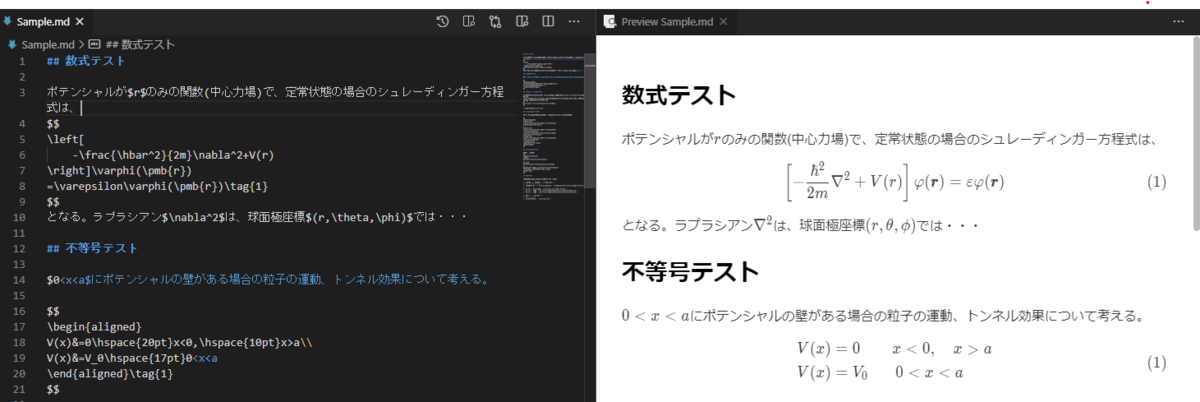VSCodeでのMPEのMarkdown編集画面とプレビュー画面。はてなブログよりかなり優秀。

これを使えば数式を含むQiitaの記事をはてなブログに移植するのも楽になると思います。

Markdown Preview Enhancedについてはこちら

Visual Studio Code + markdown preview enhanced は最高なノートだと思う - Qiita

ただ、はてなブログMarkdown特有のクセには注意が必要です。たとえば、箇条書きの直後に番号付きリストをつけるとバグる、バッククォートを含むコードがコードブロックで書けないなどなど。注意しましょう。

Markdown Preview Enhancedではいくつかの数式の挿入方法がサポートされていますが、そのうち$$で囲まれたブロック数式、で囲まれたインライン数式に対応しています。しかし、 ​math e^{i\pi} = -1 ​ には対応していません。 ## 実装 もとのmdファイルをリスト化し、文章全体を標準ブロック、数式ブロックなどとブロックごとのリストに分けてます。標準ブロックについては標準ブロック内のインライン数式をre.findallですべて検索し、順次はてな流インライン数式に変換します。数式ブロックにつていもはてなブログ用の数式ブロックになるように変換します。最後に"".join()で結合し、保存しています。 数式の変換については(styledefaultを指定した場合) • ブロック数式($$で囲まれた数式)

については

1. <div align="center">[tex:]</div>で囲む
2. ]\]に置換
3. <>\lt\gtに変換

と変換します。

• インライン数式($で囲まれた数式) については 1. [tex:\displaystyle{}]で囲む 2. ^(指数)の後ろにスペースを挿入 3. _(添え字)の前後にスペースを挿入 4. \{,\},]\\{,\\},\\]に置換 5. <>\lt\gtに変換 ## 必要環境 python3.8以上。pathilibモジュールを使います。 ## 参考サイト 以下のサイトを参考にさせていただきました。ありがとうございました。 はてなブログで数式を書く - 七誌の開発日記 Latexをはてなブログmarkdown形式に変換 - ano3のブログ # 射影平面をいくつかの方法で図示してみる # 射影平面をいくつかの方法で図示してみる こんにちはSolKulです。 今、この参考書で多視点幾何学について勉強しています。 Multiple View Geometry in Computer Vision Second Edition この参考書のPrefaceの1.1での射影幾何学射影平面についての説明が出てきますが、最初読んだとき訳が分かりませんでした。分かりにくい原因は幾何学の理論を図なしで言葉だけで説明しようとしているためだと思われます。その後いろいろ調べ、射影空間のうち二次元射影空間である射影平面について、いくつかの方法で図示できることがわかったのでメモしておきます。 ## 射影空間の導入 普段我々が慣れ親しんでいる空間の取り扱い方は、ユークリッド幾何学と呼ばれます。平面上の座標を$(x,y)$と2つの数で表すようなやり方です。しかし、このユークリッド幾何学には一つ大きな欠点があります。それは「2つの直線は1点で交わる」という法則に例外を作らないといけないという点です。その例外というのは平行です。平行な直線同士はどこまで行っても交わりません。 例外を回避するために、平行な直線は無限遠の点で交わると定義すればいいかもしれませんが、今度は無限遠の点が厳密に定義できません。このようなユークリッド幾何学の欠点を解決するために、ユークリッド空間に加えて無限遠の点も考慮する空間を考えます。これを射影空間と呼びます。 具体的には、通常、ユークリッド2次元空間上の点は$(x,y)$で表せられますが、射影空間ではそこに1つ座標を加えて$(x,y,1)$と表すことにします。また、3つの数の組で表せられる射影空間の座標は定数倍しても同じものを表すと定義します。つまり$(x,y,1)(2x,2y,2)$も同じ点を表します。数学的に正式には、「定数倍した3つの数の組の座標同士が同値であるとしたとき、ユークリッド平面の点はの3つの数の組の座標の同値類(equivalent class)で表せられる」と言います。このような座標のことを同次座標(homogeneous coordinate)と呼びます。$(x,y,1)$は最後の$1$を除くことでいつでも$(x,y)$ともとのユークリッドの表現に戻すことができますが、$(x,y,0)$についてはどうでしょう？これに対応するもとのユークリッドの座標は存在しません。もし、最後の3つの目の座標で、前2つの座標を割ろうとすれば、$(x/0,y/0)$となり、これは無限になります。こうして考慮に入れたかった無限遠の点が現れてくのです。 以上のことはどんな次元でも同様で、ユークリッド空間$\mathbb{R} ^ n$は、点を同次ベクトルとして表すことで、射影空間$\mathbb{P} ^ n$に拡張する事ができます。また、先程出てきた無限遠点は$\mathbb{P} ^ 2$では直線となることが明らかになります。それを無限遠直線(The line at infinity)と呼びます。また、3次元射影空間$\mathbb{P} ^ 3$では無限遠点は無限遠平面(The plane of infinity)となります。 ## 射影平面の図示 射影平面$\mathbb{P} ^ 2$ユークリッド平面を拡張した概念なので、図示の仕方は1通りではありません。ここではいくつかの図示の方法を示します。 ### 平らな地面を撮った写真 ユークリッド空間にとって無限遠点は特別な存在でしたが、射影空間では無限遠点も考慮に入れているので、なんら特別でなく、すべての点は等しく扱われます。また平行線というのは無限遠点で交わる線です。よって無限遠点が特別な点ではないので、射影空間では線の平行性の概念はありません。 平行であることは射影幾何学の概念ではないと言えます。 このことを頭に入れて、図を書いてみます。図を書く手順は、白い紙を用意し、それが無限に広がっていると想像します。これが射影平面$\mathbb{P} ^ 2$だと考えるのです。まず、 1. 紙に直線を描き、これが無限遠直線であることを宣言します。無限遠点は特別な存在ではないので、紙の上に適当に書いてもいいはずです。 2. 次に、この無限遠直線で交差する他の2本の線を描画します。それらは「無限遠直線」で出会うので、平行であると定義できます。(さっき平行の概念はないといったのに、平行を定義しているのはなんか変ですが、細かいことは気にしないようにします。) これを図示すると以下のようになります。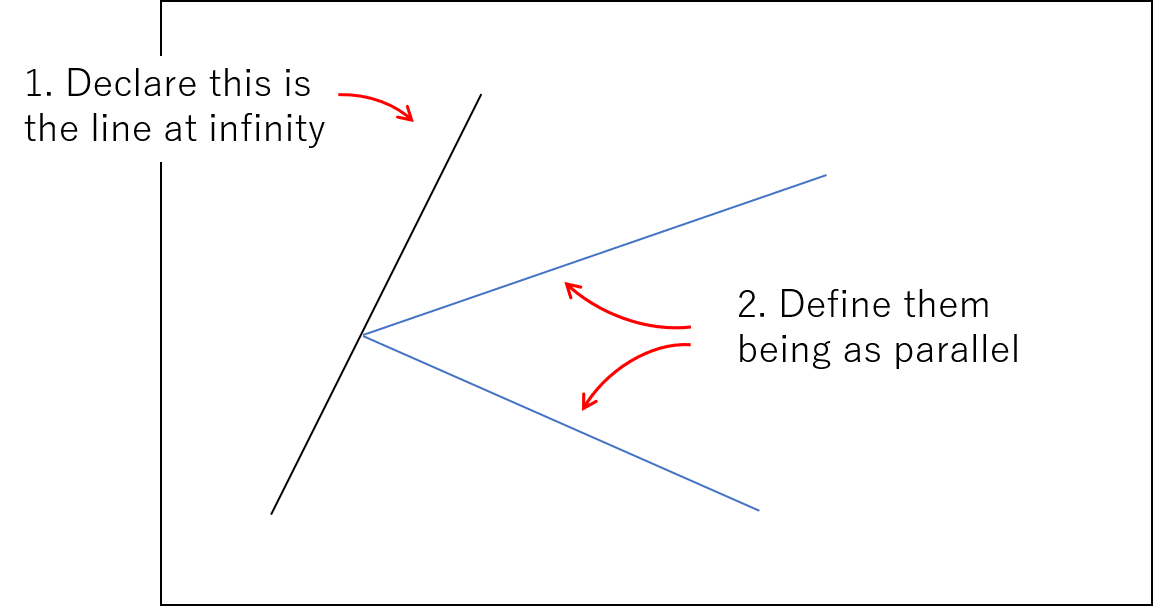射影平面の図示1:適当に無限遠直線を定義する方法 どう見ても平行ではない線を平行だと定義する、おかしな図になってしまっています。しかし皆さんはこのようなおかしな状況を1度は目にした事はあるのではないでしょうか。平行な線路が水平線上で交わっている ©MikeMalak 2006 実はこの図は平らな地面を写真を撮ったときと同じようなものです。無限遠点は水平線上に存在します。そして線路のような地面の上では平行な2つの直線は写真上では水平線上で交わるように見えます。かなり強引な解釈すれば、地面というユークリッド平面を、写真という射影平面に射影したとも言えなくもないです。 ### 平面に単純に無限遠点を加える 先程は平行でない線を平行と定義してしまいましたが、今度は平行は平行のままで図示する方法です。 平面とその周りに円を書きます。この円を無限遠直線とします。平面に平行な線を書いてみます。そしてその平行な線は無限遠点で交わるのですから、周りの円の上にその平行な直線に対応する無限遠点が一つあるはずです。別の方向の平行な直線についても対応する無限遠点が無限遠直線上にあります。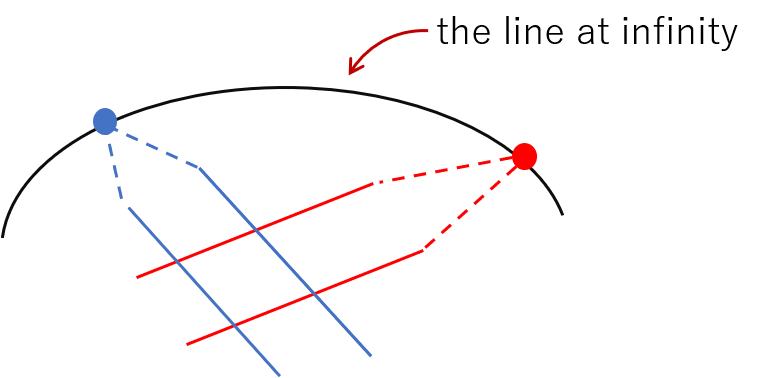射影平面の図示2:無限遠直線で囲む方法 直線が途中で無理やり折れ曲がっていたり、無限遠直線が曲がっていたりとこれも少し変な図になっています。 ### 半球を貼り合わせたもの いままでの図示では平行や無限遠点が出てきてしまっていました。本来、射影平面ではすべての点は等しく扱われ、平行という概念はないはずです。そのような図示の仕方を考えます。 半球を考えます。半球にまっすぐな線をひこうとすると、球に沿って曲がってしまいますが、半球の上に立っている小人から見ればほぼ直線です。また半球は貼り合わさられており(図示できないですが)、半球の端の点は反対側の端の点と同一視します。オレンジの直線は半球の端で反対側のオレンジの点にワープする感じです。そしてこの半球上の2つの直線は必ず一点で交わります。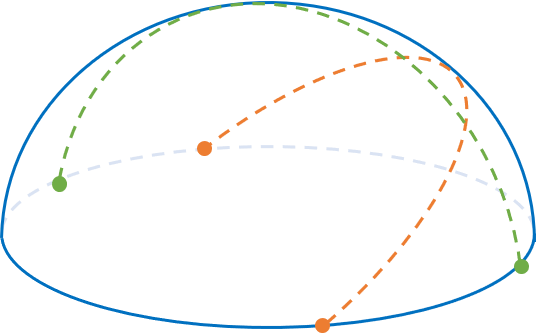射影平面の図示3:半球で考える方法 この考え方であれば、平行も無限遠点も出てこず、すべての点と直線は等しく扱えます。 ### 3次元空間で原点を通る直線 今度は、「射影空間の導入」の節で説明した同次座標をそのまま表す方法です。 まず3次元空間を考え、ユークリッド平面を$z=1$の平面に対応させます。ユークリッド平面上の点を図のように3次元空間上の原点を通る直線に対応させます。説明したように、射影平面では「定数倍した3つの数の組の座標同士が同値であるとしたとき、ユークリッド平面の点はの3つの数の組の座標の同値類(equivalent class)で表せられる」と言いました。この直線は定数倍した3つの数の組の座標の集合ですので、これがまさに同値類です。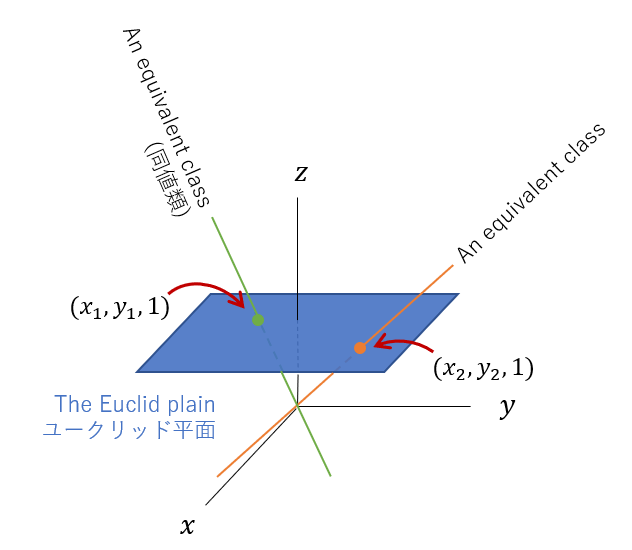射影平面の図示4:3次元空間で考える方法 そして平行とその交点はどうなるのでしょうか？まず、下図のように2つ直線の交点を考えてみます。この図示の方法ではこの交点は原点を通る直線に対応します。この2つの直線を徐々に平行に近づけていくとどうなるでしょうか？その交点は矢印の方向へ向かうように思えます。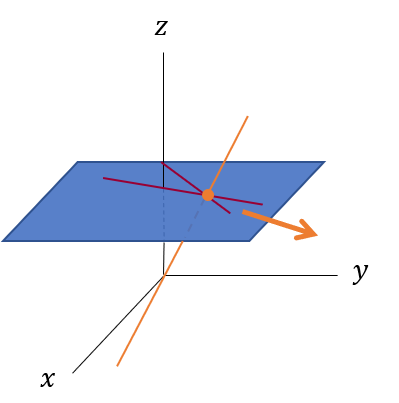2つの直線を平行に近づけていく そして最終的に2つの直線が平行になると、交点に対応する直線は、$z=0$の平面上に横倒しになるように思えます。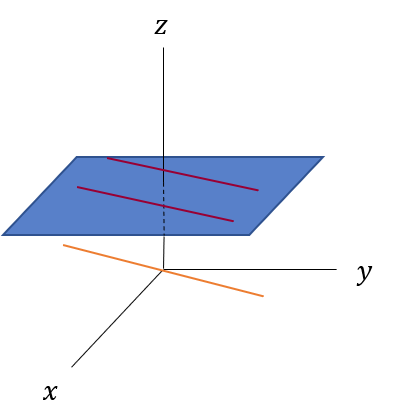ついに平行になったとき この$z=0$の平面上の直線がユークリッド平面にとっての無限遠点となります。これは同次座標の$(x,y,0)$無限遠点だと説明したこととも一致します。 そしてこの図示の方法を使えば無限遠点の集合が直線になることも説明できます。直線がユークリッド平面の点に対応するのですから、平面はユークリッド平面の直線に対応するはずです。実際、原点を通る平面をと$z=1$の平面が交差を考えればそれは直線となります。そして先ほど説明した無限遠点に対応する$z=0$の平面上の直線を集めれば、$z=0\$平面となります。平面はユークリッド平面での直線に対応するのですから、無限遠点の集合は直線、つまり無限遠直線(The line at infinity)となります。

この最後の図示の方法は同次座標との対応が取れるので厳密な議論に向いているそうです。

## 終わりに

もっと射影空間を理解したかったのなら多様体の教科書をよんだほうがいいかもしれません。私もこの記事を書いている途中でこれらの図示をする時間があればその時間を使って多様体の教科書を読んだほうがいいんじゃないかと思い始めました。しかし、個人的にきれいな図を残して知識を整理してみたかったのでこの記事を作ってみました。